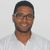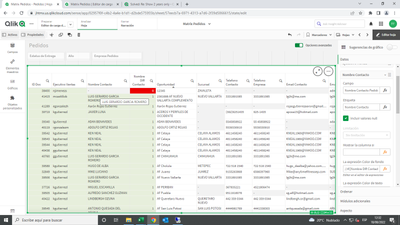# New to Qlik Sense

If you’re new to Qlik Sense, start with this Discussion Board and get up-to-speed quickly.

Announcements
QlikWorld 2023, a live, in-person thrill ride. Save \$300 before February 6: REGISTER NOW!
cancel
Showing results for
Did you mean:Contributor III

## Show 2 years only

Hi everyone,

l have a conecction to a sql server, and have data since 2015, but l only want to work with 2021 and 2022, is possible to see only that in for example a table or filter?

Set Analysis is good option?

I used set analysis in another model and that model has MasterCalendar but in this new one l dont want to use MasterCalendar and l think it has relationship for use Set Analysis

Or how can l see only that years?

Thank you all

Labels (7)

• ### Variables

2 Solutions

Accepted SolutionsMVP

irst, if you don't need the other years, you can filter from the load script the two years

for example :
load .... where year=2021 or year=2020

otherwise in set analysis you can use for your measure:

sum( {<YEAR={'2021','2022'}>}YOURMEASURE)

or you can filter the dimension with the if function for example
if(YEAR=2021 or YEAR=2022,YEAR) and delete the null value

Regards,
Taoufiq ZARRA

"Please LIKE posts and "Accept as Solution" if the provided solution is helpful "

(you can mark up to 3 "solutions") 😉Specialist II

To load only the required years into the data model try this

SQL SELECT *
FROM SQL.dbo.TABLE

Where date_field  >=  DATEADD(yy, -1, GETDATE());  //Fetching only 2021 and 2022

Alternatively, you can try the below set analysis expression

Sum({<[Year Field]={'>=\$(=max([Year Field])-2)<\$(=max([Year Field]))'}>} [Measure Field])

6 RepliesMVP

irst, if you don't need the other years, you can filter from the load script the two years

for example :
load .... where year=2021 or year=2020

otherwise in set analysis you can use for your measure:

sum( {<YEAR={'2021','2022'}>}YOURMEASURE)

or you can filter the dimension with the if function for example
if(YEAR=2021 or YEAR=2022,YEAR) and delete the null value

Regards,
Taoufiq ZARRA

"Please LIKE posts and "Accept as Solution" if the provided solution is helpful "

(you can mark up to 3 "solutions") 😉Specialist II

To load only the required years into the data model try this

SQL SELECT *
FROM SQL.dbo.TABLE

Where date_field  >=  DATEADD(yy, -1, GETDATE());  //Fetching only 2021 and 2022

Alternatively, you can try the below set analysis expression

Sum({<[Year Field]={'>=\$(=max([Year Field])-2)<\$(=max([Year Field]))'}>} [Measure Field])Contributor III
Author

Thanks to @Taoufiq_Zarra  and you for your help. It works good

Its first time l work with connection, always worked with LOAD by excel

Even l have another fast question. In my script,  l have this:

if(len( ContactName) >=1,1,0) ) as Nombre Contacto

so now in a table lm using ContactName Field, and in  color expresion using this:

if([Nombre Contacto ]<1,rgb(235,0,0),rgb(231,239,220))

but it doesnt paint Red color, only the green, and in next Column paint correctly Red Color and greenDo you know why this happend? l check in the connection exporting to excel and is Empty the field so ldk why no works good

Thank you againSpecialist II

@fernandoflogtzperhaps this

IF([Nombre Contacto ]=0,rgb(235,0,0),rgb(231,239,220))Contributor III
Author

Hi Peter

I try it and still not painting the red colorI try many ways of condition but is not workingSpecialist II

I suppose you can refer to the expression Name rather than using the actual expression.

In short,  your expression name is "Nombre DIR Contacto" so the colour expression perhaps this

IF((([Nombre DIR Contacto] = 0, rgb(235,0,0)
and [Nombre DIR Contacto] = 1,rgb(231,239,220))

Hope this helps you.Tags
Community Browser